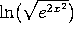M403K Second Midterm Exam
March 7, 2002

Problem 1. Graphing (2 pages)

Consider the function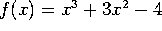. (This function factors as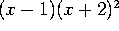).

a) Find the partition points of f (if there are any) and make a sign chart for f.

b) Find the critical points of f (if there are any) and make a sign chart for f'.

c) Find the inflection points of f (if there are any) and make a sign chart for f''.

d) Sketch the graph of the function. Show clearly the location of the partition points, critical points and inflection points, and the value of the function at these special points. Be sure that the positivity, direction, and curvature of your graph agree with your calculations in parts a-c.

Problem 2. Max-min

Find the absolute maximum and absolute minimum values of the function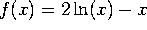on the interval [1,3].

Problem 3. Wild West WIMPs

Widget Industries of Missoula and Pocatello (WIMP) is offering high-tech digital widgets to their customers in Montana and Idaho. They estimate that the demand x is related to the price p by the demand equation x=100-p. Their cost function is C(x)=40x+600.

a) Write down the revenue R(x) and the profit P(x) as functions of x.

b) Find the value of x that maximizes revenue. At that production level, what will the price be? How much revenue will the company bring in?

c) Find the value of x that maximizes profit? How big is that maximum profit?

Problem 4. Implicit differentiation

Consider the curve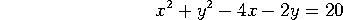.

a) Find dy/dx at the point (x,y).

b) Find the slope of the line tangent to the curve at the point (5,5).

c) Estimate the value of y when x=5.04.

Problem 5. Exponential Growth

A piece of cheese left in the refrigerator too long has become a biology experiment. The mold on it is growing exponentially, with a growth rate of 10%/day (compounded continuously!). Currently there are 3 grams of mold.

a) How much mold will there be in x days? (Write down a formula, with all the numbers except x filled in).

b) How long will it take for the mold to double?

c) When will there be 24 grams of mold on the cheese? Simplify your answer as much as possible.

Problem 6. Exponentials and logs

For a, b, and c, take the derivatives, with respect to x, of the following functions. Your final answer should be expressed entirely in terms of x. (e.g.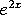, not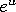). However, in these parts you do NOT need to simplify your results further.

a)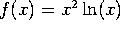b)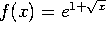c)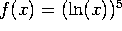d) Simplify the expression